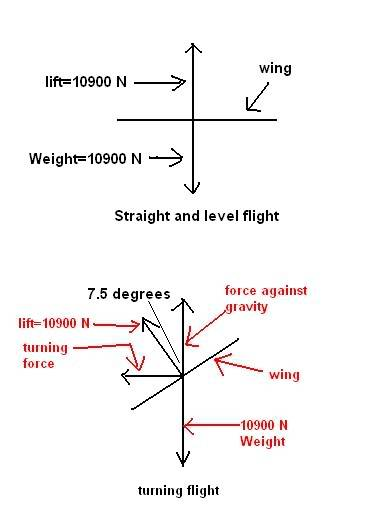# Airplane Free-body diagram

• ataglance05
In summary, we discuss the free-body diagram of a plane in level flight and calculate the force of gravity and lifting force. We then introduce a turn by banking the wings at a 7.5 degree angle, which decreases the lifting force and allows the plane to turn in a circle without descending. We are asked to calculate the time it takes for the plane to make one turn at a constant velocity of 200 km/hr. Using the formula Time=Distance/Velocity and the circumference of the circle, we can find the time it takes for the plane to complete the turn.

#### ataglance05

Airplane Free-body diagram!

## Homework Statement

A plane with mass of 1090 kg is flying straight and level at an altitude of 1100 meters & a constant velocity of 200 kg/hr (55.55m/sec). Assuming that the acceleration due to gravity is 10 m/sec^2, the force on the plane due to gravity is 10900 Newtons. Since the plane is in level flight, the net force in the y direction is 0 & the lifting force provided by the wings equals the weight of the plane. The piolot now banks the wings at a 7.5 degree angle w/ respect to the vertical in order to make a turn. Part of the lifting force of the wings is now used to make the plane turn in a circle & there's less lift to counteract the force of gravity.

How long will it take the plane to make one turn of 360 degrees at a velocity of 200 km/hr (55.55 m/sec)??

## Homework Equations

Vf=Vo+aT
S=Vo(T)+1/2(a)(T)^2
s=theta(r)
A free-body diagram of the situation:## The Attempt at a Solution

NO CLUE! HELP!

All I know is that the value that the lift should be increased so that the plane doesn't decend is 10994 Newtons and that the radius of the circle that the plane will fly w/o loosing altitude w/ constant velocity of 200km/hr is 2343 m.

Last edited:
anyone?Well, if you know the radius of the circle, you can easily find the circumference of the circle. What does that give you? You also know the plane's speed. You can put these two pieces of information together to find out the time it takes to complete the turn.

hage567 said:
Well, if you know the radius of the circle, you can easily find the circumference of the circle. What does that give you? You also know the plane's speed. You can put these two pieces of information together to find out the time it takes to complete the turn.

The circumference is 7326.194 and the velocity is 55.55 m/sec. what equation would i use though??

Double check your calculation for the circumference, I got a different value. You know the circumference (ie total distance) the plane travels in one turn, and the speed during the turn. What's the definition of speed? Use that and solve for t.

hage567 said:
Double check your calculation for the circumference, I got a different value. You know the circumference (ie total distance) the plane travels in one turn, and the speed during the turn. What's the definition of speed? Use that and solve for t.

I stand corrected; C=14714.04

Alright so I'm now leaning towards Time=Distance/Velocity with the distance being the circumference. Am i correct?

Sounds good to me!

thanks!